# Pion annihilation Feynman diagram

π+ + π- → γ + γ

How do you represent this in a Feynman diagram showing the individual quark? I am very confused please help!

[Moderator's note: Moved from a technical forum and thus no template. Own effort below.]

Last edited by a moderator:

Do you know what the pions are made up of? If you don't, look it up. (both have 2 quarks)

mathman
Are you playing a game?

•Replusz
Do you know what the pions are made up of? If you don't, look it up. (both have 2 quarks)
Yes, the pi plus is an up and an anti down, and anti up and down for the minus. The only way I can think to draw that is with the down and anti down annihilating into a photon and same for the up and anti up? I'm not sure if there's a way to do it as one diagram?

•vanhees71
mfb
Mentor
That is one diagram.
You can connect some quarks with a gluon if you like.

That is one diagram.
You can connect some quarks with a gluon if you like.

Um, so far I have this? Is this what you mean?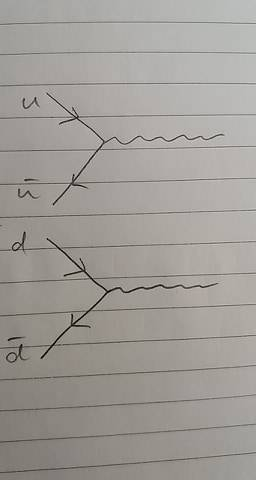mfb
Mentor
I would start the diagram with the individual pions as groups (first the two quarks of the pi-, then the two quarks of the pi+, or vice versa), even though that means two lines have to cross.

I would start the diagram with the individual pions as groups (first the two quarks of the pi-, then the two quarks of the pi+, or vice versa), even though that means two lines have to cross.

Right. Where the lines cross, I assumed another interaction happens? I found this while on a googling rampage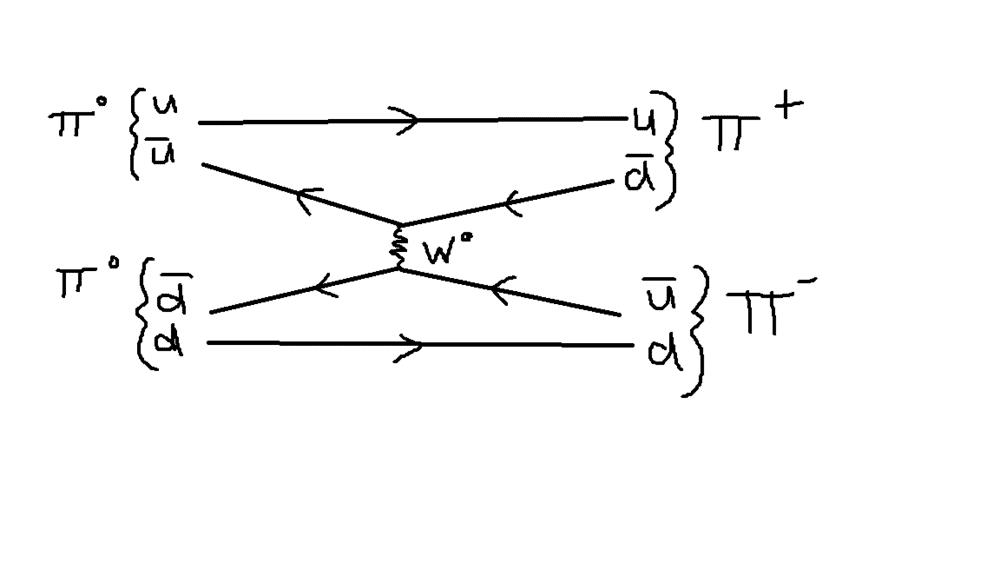from https://physics.stackexchange.com/questions/235366/feynman-diagram-pi0-pi0-rightarrow-pi-pi
If this person is right (I am far too inexperienced to tell that as is clear!) then by rotating this through 180° gets another valid diagram. Now, if this is all true, can the quark-antiquark pairs annihilate into a photon each, or do the π0s decay into two photons each like this?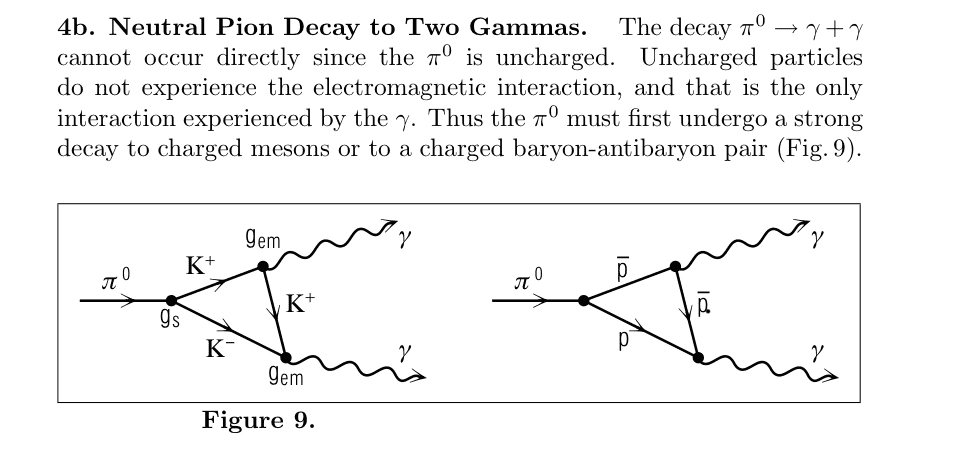Of course the other option is I have overcomplicated this horrendously, but from this fudge of a situation I have this: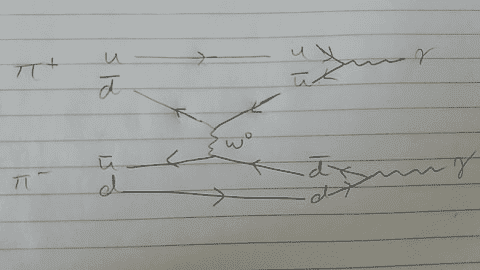Thank you for your patiencemfb
Mentor
Where the lines cross, I assumed another interaction happens?
No. The position of the lines is largely arbitrary and crossing lines don't mean anything.

There is no weak interaction here.

•Physicsphysics
Staff Emeritus
How do you represent this in a Feynman diagram showing the individual quark?

Why do you want this? A Feynman diagram is a calculational tool, and this calxulation is much easier in terms of pions than quarks. (Probably not even possible with quarks, at least not if you want to get the right answer)

Last edited:
Why do you want this? A Feynman diagram is a calculational tool, and this xalxulation is much easier in terms of pions than quarks. (Probably not even possible with quarks, at least not if you want to get the right answer)
This is a task set by my professor and we're meant to show the quarks explicitly. We've been taught the basic rules with Feynman diagrams but honestly not much of the maths so this is almost entirely guesswork. It is quite frustrating with the hilariously superficial "understanding" I have atm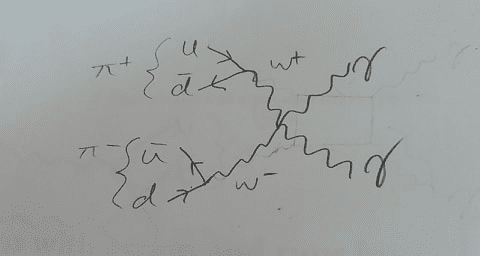After some discussion, this was the conclusion... Is this correct?

Staff Emeritus
After some discussion, this was the conclusion... Is this correct?
There is no weak interaction here.

Are you reading what we write?

Are you reading what we write?

mfb
Mentor
There is no weak interaction here.

Dr_Nate